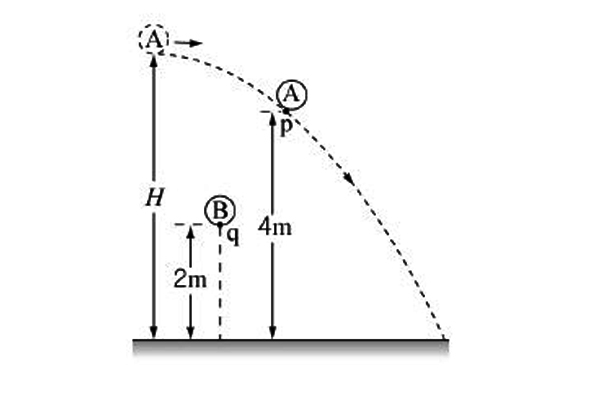# Gravitational accelerationObject $A$ is thrown horizontally from a height of $H.$ At the moment it passes point $p$ which is $4$ m above the ground, object $B$ is dropped from point $q$ which is $2$ m above the ground. If both objects touch the ground at the same time, what is $H$ (in m)?

Gravitational acceleration is $g = 10$ m/s$^{2}.$

×

Problem Loading...

Note Loading...

Set Loading...